Download Presentation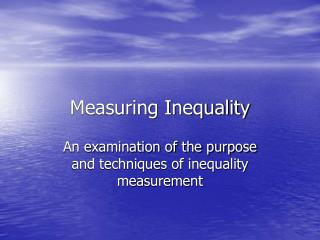Measuring Inequality

# Measuring Inequality - PowerPoint PPT Presentation

Measuring Inequality. An examination of the purpose and techniques of inequality measurement. What is inequality?. From Merriam-Webster:.I am the owner, or an agent authorized to act on behalf of the owner, of the copyrighted work described.
Download Presentation## Measuring Inequality

An Image/Link below is provided (as is) to download presentation

Download Policy: Content on the Website is provided to you AS IS for your information and personal use and may not be sold / licensed / shared on other websites without getting consent from its author.While downloading, if for some reason you are not able to download a presentation, the publisher may have deleted the file from their server.

- - - - - - - - - - - - - - - - - - - - - - - - - - E N D - - - - - - - - - - - - - - - - - - - - - - - - - -
Presentation Transcript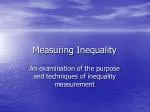### Measuring Inequality

An examination of the purpose and techniques of inequality measurement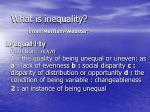What is inequality?

From Merriam-Webster:

in·equal·i·tyFunction: noun1: the quality of being unequal or uneven: as a: lack of evenness b: social disparity c: disparity of distribution or opportunity d: the condition of being variable : changeableness

2: an instance of being unequal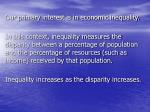Our primary interest is in economic inequality.

In this context, inequality measures the disparity between a percentage of population and the percentage of resources (such as income) received by that population.

Inequality increases as the disparity increases.If a single person holds all of a given resource, inequality is at a maximum. If all persons hold the same percentage of a resource, inequality is at a minimum. Inequality studies explore the levels of resource disparity and their practical and political implications.Economic Inequalities can occur for several reasons:

• Physical attributes – distribution of natural ability is not equal
• Personal Preferences – Relative valuation of leisure and work effort differs
• Social Process – Pressure to work or not to work varies across particular fields or disciplines
• Public Policy – tax, labor, education, and other policies affect the distribution of resources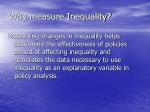Why measure Inequality?

Measuring changes in inequality helps determine the effectiveness of policies aimed at affecting inequality and generates the data necessary to use inequality as an explanatory variable in policy analysis.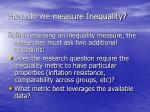How do we measure Inequality?

Before choosing an inequality measure, the researcher must ask two additional questions:

• Does the research question require the inequality metric to have particular properties (inflation resistance, comparability across groups, etc)?
• What metric best leverages the available data?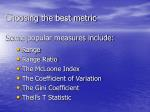Choosing the best metric
• Range
• Range Ratio
• The McLoone Index
• The Coefficient of Variation
• The Gini Coefficient
• Theil’s T Statistic

Some popular measures include: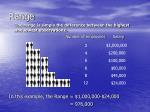Range

The range is simply the difference between the highest and lowest observations.

Number of employees

Salary

\$1,000,000

2

\$200,000

4

6

\$100,000

6

\$60,000

8

\$45,000

12

\$24,000

In this example, the Range = \$1,000,000-\$24,000

= 976,000Pros

Easy to Understand

Easy to Compute

Cons

Ignores all but two of the observations

Does not weight observations

Affected by inflation

Skewed by outliers

Range

The range is simply the difference between the highest and lowest observations.Range Ratio

The Range Ratio is computed by dividing a value at one predetermined percentile by the value at a lower predetermined percentile.

Salary

Number of employees

95 percentile

Approx. equals

36th person

\$1,000,000

2

\$200,000

4

6

\$100,000

5 percentile

Approx. equals

2nd person

6

\$60,000

8

\$45,000

12

\$24,000

In this example, the Range Ratio=200,000/24,000 =8.33

Note: Any two percentiles can be used in producing a Range Ratio. In some contexts, this 95/5 ratio is referred to as the Federal Range Ratio.Pros

Easy to understand

Easy to calculate

Not skewed by severe outliers

Not affected by inflation

Cons

Ignores all but two of the observations

Does not weight observations

Range Ratio

The Range Ratio is computed by dividing a value at one predetermined percentile by the value at a lower predetermined percentile.The McLoone Index

The McLoone Index divides the summation of all observations below the median, by the median multiplied by the number of observations below median.

Number of employees

Salary

1,000,000.00

2

200,000.00

4

6

100,000.00

Observations

below

median

6

60,000.00

8

45,000.00

12

24,000.00

In this example, the summation of observations below the

median = 603,000, and the median = 45,000

Thus, the McLoone Index = 603,000/(45,000(19)) = .7053Pros

Easy to understand

Conveys comprehensive information about the bottom half

Cons

Ignores values above the median

Relevance depends on the meaning of the median value

The McLoone Index

The McLoone Index divides the summation of all observations below the median, by the median multiplied by the number of observations below median.The Coefficient of Variation

The Coefficient of Variation is a distribution’s standard deviation divided by its mean.

Both distributions above have the same mean, 1, but the standard deviation is much smaller in the distribution on the left, resulting in a lower coefficient of variation.Pros

Fairly easy to understand

If data is weighted, it is immune to outliers

Incorporates all data

Not skewed by inflation

Cons

Requires comprehensive individual level data

No standard for an acceptable level of inequality

The Coefficient of Variation

The Coefficient of Variation is a distribution’s standard deviation divided by its mean.The Gini Coefficient

The Gini Coefficient has an intuitive, but possibly unfamiliar construction.

To understand the Gini Coefficient, one must first understand the Lorenz Curve, which orders all observations and then plots the cumulative percentage of the population against the cumulative percentage of the resource.The Gini Coefficient

An equality diagonal represents perfect equality: at every point, cumulative population equals cumulative income.

• A – Equality Diagonal Population = Income
• B – Lorenz Curve
• C – Difference Between Equality and Reality

The Lorenz curve measures the actual distribution of income.

CumulativeIncome

A

C

B

Cumulative PopulationThe Gini Coefficient

Mathematically, the Gini Coefficient is equal to twice the area enclosed between the Lorenz curve and the equality diagonal.

When there is perfect equality, the Lorenz curve is the equality diagonal, and the value of the Gini Coefficient is zero.

When one member of the population holds all of the resource, the value of the Gini Coefficient is one.Pros

Generally regarded as gold standard in economic work

Incorporates all data

Allows direct comparison between units with different size populations

Attractive intuitive interpretation

Cons

Requires comprehensive individual level data

Requires more sophisticated computations

The Gini Coefficient

Twice the area between the Lorenz curve and the equality diagonal.Theil’s T Statistic

Theil’s T Statistic lacks an intuitive picture and involves more than a simple difference or ratio.

Nonetheless, it has several properties that make it a superior inequality measure.

Theil’s T Statistic can incorporate group-level data and is particularly effective at parsing effects in hierarchical data sets.Theil’s T Statistic

Theil’s T Statistic generates an element, or a contribution, for each individual or group in the analysis which weights the data point’s size (in terms of population share) and weirdness (in terms of proportional distance from the mean).

When individual data is available, each individual has an identical population share (1/N), so each individual’s Theil element is determined by his or her proportional distance from the mean.Theil’s T Statistic

Mathematically, with individual level data Theil’s T statistic of income inequality is given by:

where n is the number of individuals in the population, ypis the income of the person indexed by p, and µyis the population’s average income.Theil’s T Statistic

The formula on the previous slide emphasizes several points:

• The summation sign reinforces the idea that each person will contribute a Theil element.
• yp/µy is the proportion of the individual’s income to average income.
• The natural logarithm of yp /µy determines whether the element will be positive (yp /µy > 1); negative (yp /µy < 1); or zero (yp /µy = 0).Theil’s T Statistic – Example 1

The following example assumes that exact salary information is known for each individual.

Number of employees

Exact Salary

\$100,000

2

\$80,000

4

6

\$60,000

4

\$40,000

2

\$20,000

For this data, Theil’s T Statistic = 0.079078221

Individuals in the top salary group contribute large positive elements. Individuals in the middle salary group contribute nothing to Theil’s T Statistic because their salaries are equal to the population average. Individuals in the bottom salary group contribute large negative elements.Theil’s T Statistic

Often, individual data is not available. Theil’s T Statistic has a flexible way to deal with such instances.

If members of a population can be classified into mutually exclusive and completely exhaustive groups, then Theil’s T Statistic for the population (T ) is made up of two components, the between group component (T’g) and the within group component (Twg).Theil’s T Statistic

Algebraically, we have:

T = T’g + Twg

When aggregated data is available instead of individual data, T’gcan be used as a lower bound for Theil’s T Statistic in the population.Theil’s T Statistic

The between group element of the Theil index has a familiar form:

where i indexes the groups, pi is the population of group i, P is the total population, yiis the average income in group i, and µ is the average income across the entire population.Theil’s T Statistic – Example 2

Now assume the more realistic scenario where a researcher has average salary information across groups.

Number of employees in group

Group Average Salary

2

\$95,000

\$75,000

4

6

\$60,000

4

\$45,000

2

\$25,000

For this data, T’g= 0.054349998

The top salary two salary groups contribute positive elements. The middle salary group contributes nothing to the between group Theil’s T Statistic because the group average salary is equal to the population average. The bottom two salary groups contribute negative elements.Group analysis with Theil’s T Statistic:

As Example 2 hints, Theil’s T Statistic is a powerful tool for analyzing inequality within and between various groupings, because:

• The between group elements capture each group’s contribution to overall inequality
• The sum of the between group elements is a reasonable lower bound for Theil’s T statistic in the population
• Sub-groups can be broken down within the context of larger groupsPros

Can effectively use group data

Allows the researcher to parse inequality into within group and between group components

Cons

No intuitive motivating picture

Cannot directly compare populations with different sizes or group structures

Comparatively mathematically complex

Theil’s T StatisticNext Steps
• Those interested in a more rigorous examination of inequality metrics with several numerical examples should proceed to The Theoretical Basics of Popular Inequality Measures.
• Otherwise, proceed to A Nearly Painless Guide to Computing Theil’s T Statistic which emphasizes constructing research questions and using a spreadsheet to conduct analysis.